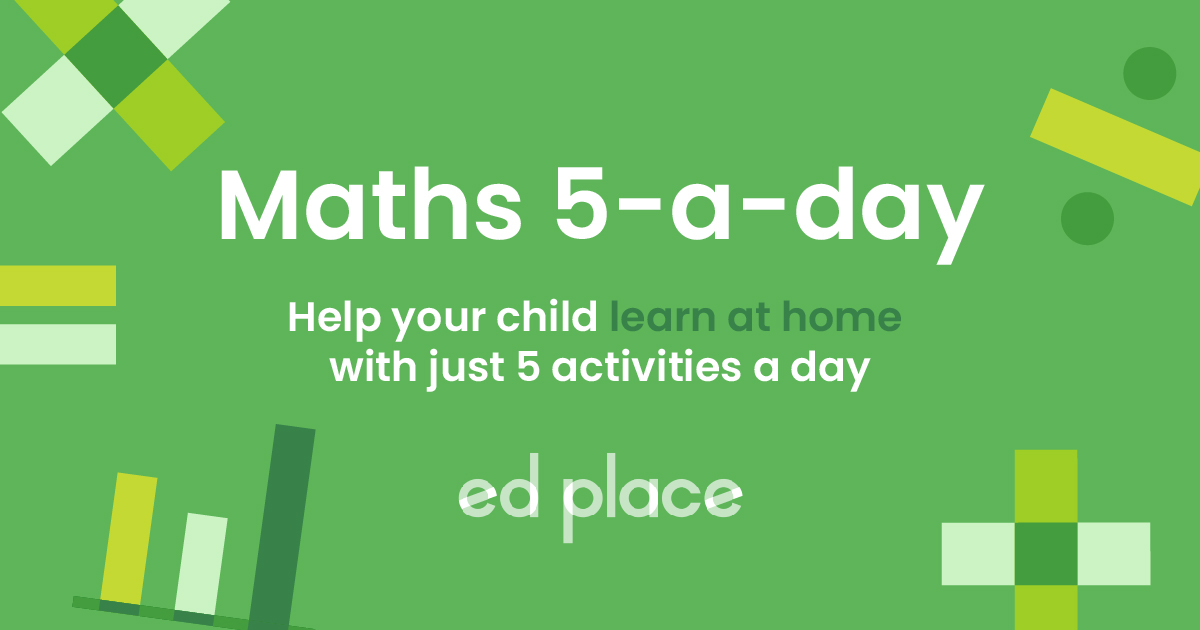# EdPlace's Year 1 and 2 Home Learning Maths Lesson: Find Missing Numbers in Addition and Subtraction Sentences

Looking for short lessons to keep your child engaged and learning? Our experienced team of teachers have created English, maths and science lessons for the home, so your child can learn no matter where they are.  And, as all activities are self-marked, you really can encourage your child to be an independent learner.

Get them started on the lesson below and then jump into our teacher-created activities to practice what they've learnt. We've recommended five to ensure they feel secure in their knowledge - 5-a-day helps keeps the learning loss at bay (or so we think!).

Are they keen to start practising straight away? Head to the bottom of the page to find the activities.

Now...onto the lesson!

Key Stage 1 Statutory Requirements for Maths
Years 1 and 2 students should be able to solve missing number problems such as 7 = ? - 9 and recognise and use the inverse relationship between addition and subtraction to check calculations and solve missing number problems.

Did you know that your child won't only learn the concept of sentences in English, but also in their maths lessons too?  More specifically, they'll be faced with an addition or subtraction sentence with a missing number, in other words, what you may also know as a sum. The question is, how do you they find the missing number using the most simple and effective method? As a parent, you may well be wondering the best way to explain this in a child-friendly way so that you're child really grasps this concept?

Follow the step-by-step guide below to clarify how to solve number sentences with your child.  By the end of this article we're confident that your child will be able to:

1)  Understand how to find the missing number in an addition or subtraction sentence

2) Apply this understanding to describe the inverse relationship needed to solve the problem

3) Explain it back to you at the end!

## Step 1: The Key Terms

Before we get going, it's crucial to check your child understands some important language we will use to complete our lesson objectives.  These words form the foundations for all maths skills, so mastering these definitions early will really help your child to make progress.

Subtraction: the ‘taking away’ of one number from another to make the original number smaller. Think of ‘subtracting’ as taking apart.

Inverse relationship: this specifically looks at how the relationship between addition and subtraction reverses the effect of the other operation.

## Step 2: Further Explanation into Key Concepts

To tackle this lesson, your child will also need a sound understanding of these key concepts:

Addition: Adding two or more given numbers together to find a total, we can call this the total sum of the numbers. Initially, your child will have been taught to add using concrete objects and will then have progressed onto using a number line.  If they are struggling, see what you can find in the house to make this visual.  We find that food items can be quite a motivating tool here!

Subtraction: Subtracting allows us to find the difference between two values. Reinforce with your child that we must always subtract the smallest number from the largest. Again, your child will have been taught to subtract using concrete objects and will then have progressed onto using a number line to count backwards.

Number bonds: This is the concept of pairs of numbers that go together to make a number.  If we take 10 as the answer we are looking for, we find that the pairs we have are; (1, 9) (2, 8) (3, 7) (4, 6) (5 ,5) If your child knows number bonds well, it will be easy for them to use the inverse to solve a question such as 10 - ? = 3 as they will quickly recall that 7 + 3 = 10.

## Step 3: Understand the Inverse Relationship Between Addition and Subtraction

To find a missing number in an addition or subtraction sentence, we must understand the inverse relationship between addition and subtraction.

Inverse means the opposite.  It can sound like a complicated word to children so make sure to keep reinforcing this point.

For example: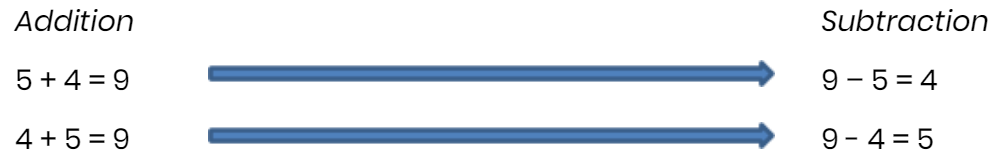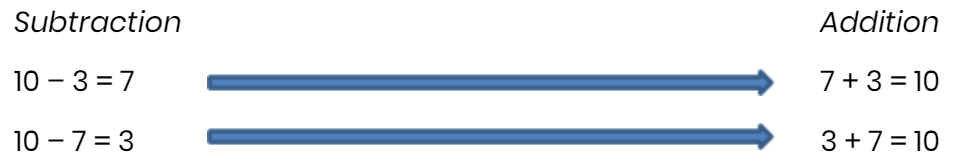1) If there is a missing number at the beginning of the sentence, do the inverse!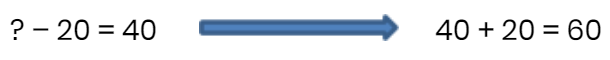Check: 60 - 20 = 40

2) If there is a missing number in the middle of the sentence, do a subtraction, using the numbers you have been given to solve it!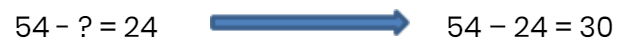Check: 54 - 30 = 24

## Step 4 - Put it into Practice

Now, have a go at using the rules to solve these sums together.

1) ? – 14 = 6

2) 11 + ? =20

3) ? + 32 = 50

4) 92 - ? = 54

## Step 5 - Give it a go...

Great effort covering all this together! Now, why not challenge your child further by assigning the following 5 activities in this order?

All activities are created by teachers and automatically marked. Plus, with an EdPlace subscription, we can automatically progress your child at a level that's right for them. Sending you progress reports along the way so you can track and measure progress, together - brilliant!

1) 20 – 14 = 6

2) 11 + 9 = 20

3) 18 + 32 = 50

4) 92 – 38 = 54

Keep going! Looking for more activities, different subjects or year groups?

Click the button below to view the EdPlace English, maths, science and 11+ activity library